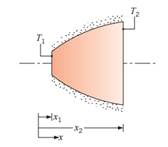### Create an Account

Home / Questions / The diagram shows a conical section fabricated from pure aluminum It is of circular cross ...

# The diagram shows a conical section fabricated from pure aluminum It is of circular cross section having diameter D ax12 where a 05 m12 The small end is located at x1 25 mm and the large

The diagram shows a conical section fabricated from pure aluminum. It is of circular cross section having diameter D = ax1/2, where a = 0.5 m1/2. The small end is located at x= 25 mm and the large end at x2 = 125 mm. The end temperatures are T1 = 600 K and T2 = 400 K, while the lateral surface is well insulated.(a) Derive an expression for the temperature distribution T(x) in symbolic form, assuming one-dimensional conditions. Sketch the temperature distribution.

(b) Calculate the heat rate qx.

Jun 28 2020 View more View LessSubscribe To Get Solution